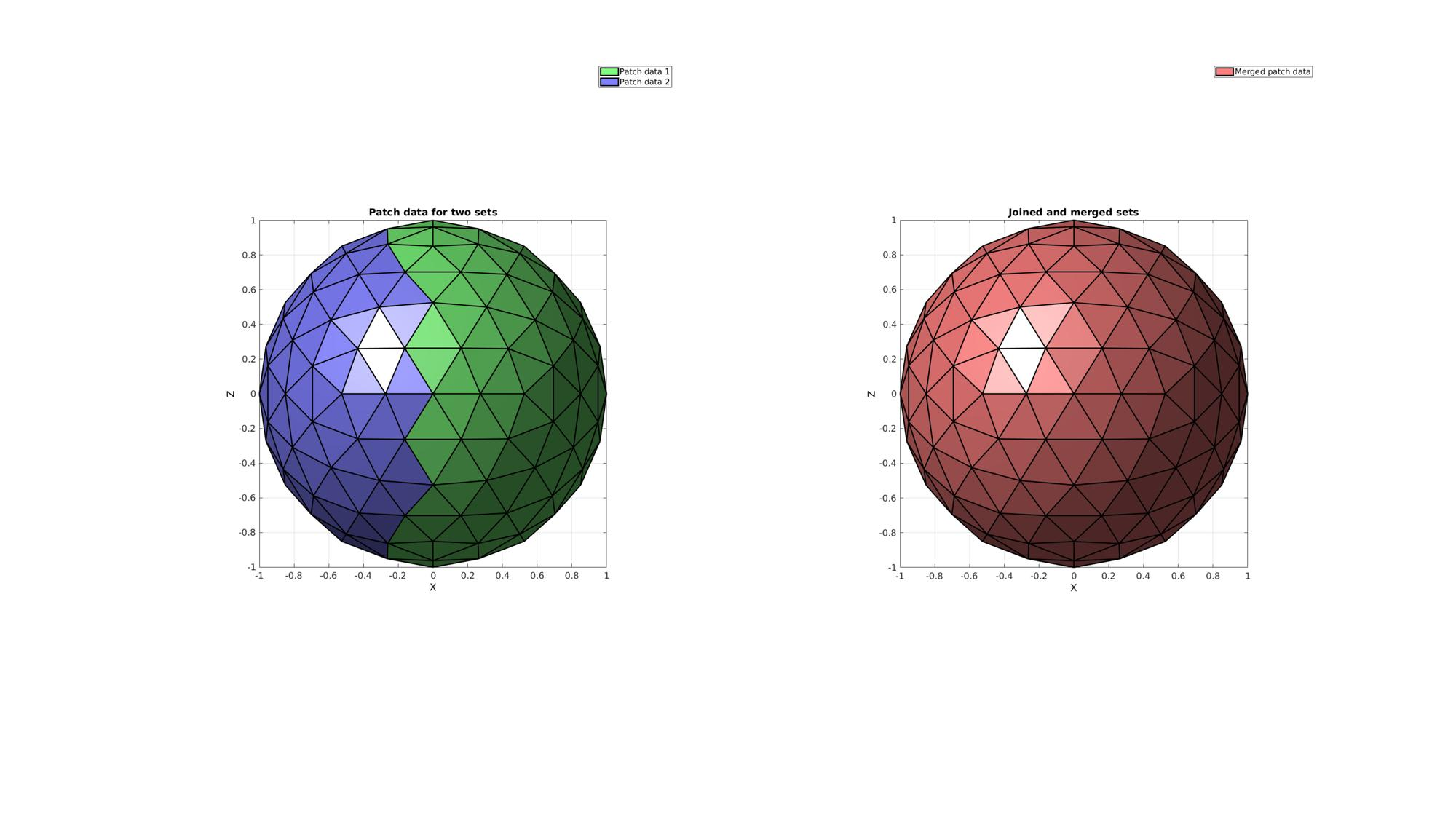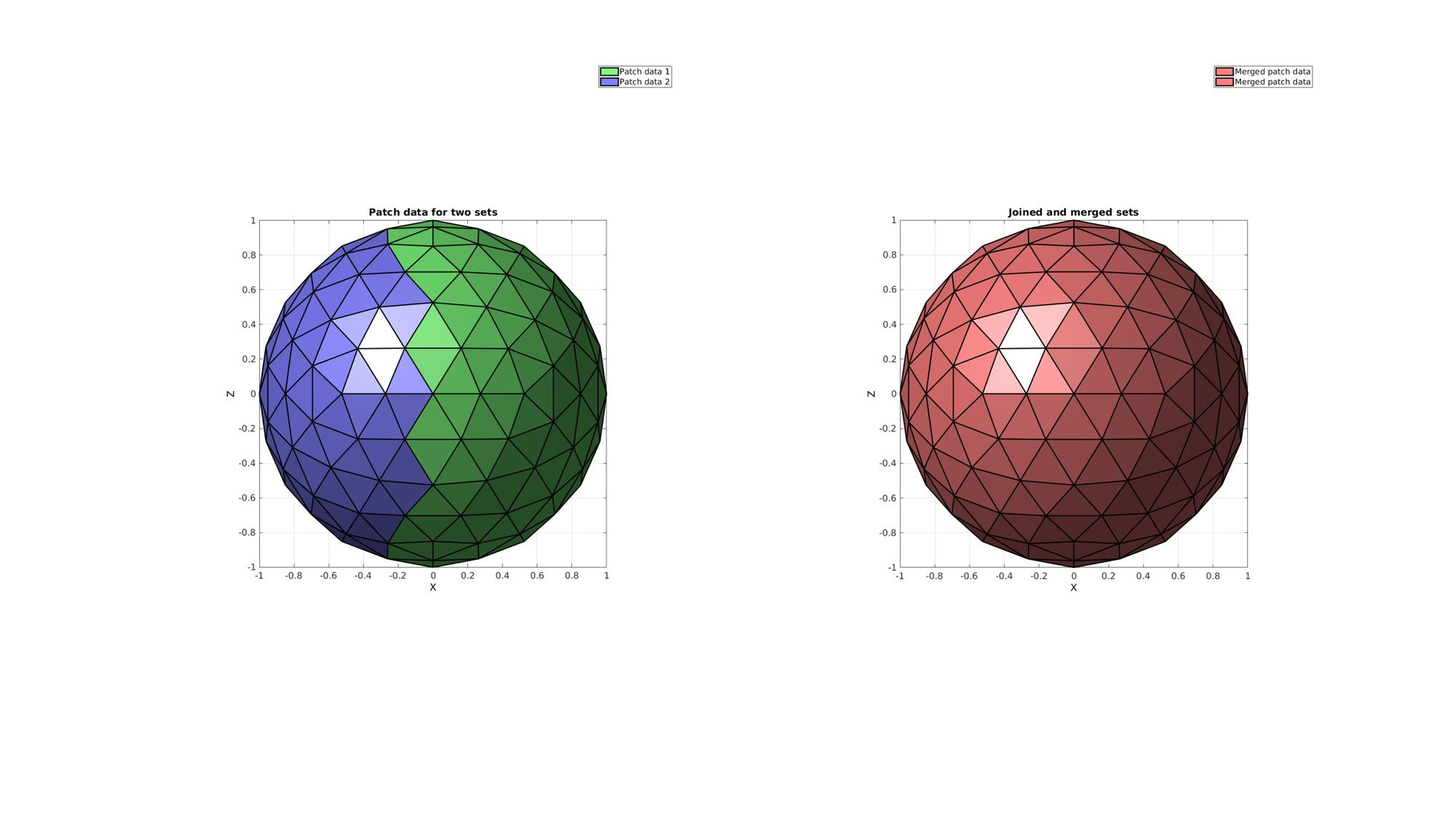# mergeVertices

Below is a demonstration of the features of the mergeVertices function

## Syntax

[F,V,ind1,ind2]=mergeVertices(F,V,numDigitsMerge);

## Description

Merges vertices that are deemed the in terms of a certain number of significant digits

## Examples

```clear; close all; clc;
```

Plot settings

```fontSize=15;
plotColor1=0.25.*ones(1,3);
plotColor2=0.75.*ones(1,3);
edgeWidth=2;
markerSize=30;
```

## Example 1: Merging vertices in patch data

Creating example patch data with unused nodes. This example is a sphere triangulation. The sphere is roughly cut in half by deleting half of its triangles. However, after the cut operation the nodes which used to be used by the deleted half are still present in the vertex array.

Defining geodesic dome triangulation

```r=1; %sphere radius
n=2; %Refinements
[Fs,Vs]=geoSphere(n,r); %The sphere triangulation
```

Cropping away a selection of faces

```logic1Vertices=Vs(:,1)>0; %Logic on vertices
logic1=any(logic1Vertices(Fs),2); %Logic on faces
```

Removing unused nodes using patchCleanUnused

```[F1,V1]=patchCleanUnused(Fs(logic1,:),Vs);
[F2,V2]=patchCleanUnused(Fs(~logic1,:),Vs);

% Joining node sets (without merging)
[F,V]=joinElementSets({F1,F2},{V1,V2});
```

Merging shared nodes using mergeVertices

```size(V)
[F,V]=mergeVertices(F,V);
size(V)
```
```ans =

184     3

ans =

162     3

```

Visualize results

```cFigure;
subplot(1,2,1); hold on;
title('Patch data for two sets');
hp(1)=gpatch(F1,V1,'gw','k',1,edgeWidth);
hp(2)=gpatch(F2,V2,'bw','k',1,edgeWidth);
legend(hp,{'Patch data 1','Patch data 2'});
axisGeom(gca,fontSize);
view(0,0); ha=axis;

subplot(1,2,2); hold on;
title('Joined and merged sets');
clear hp;
hp(1)=gpatch(F,V,'rw','k',1,edgeWidth);
legend(hp,{'Merged patch data'});
axisGeom(gca,fontSize);
view(0,0); axis(ha);
drawnow;
```## Example 2: Merging nodes on a cell array of meshes

```F={F1,F2+size(V1,1)};
V=[V1;V2];
```

Merging shared nodes using mergeVertices

```size(V)
[F,V]=mergeVertices(F,V);
size(V)
```
```ans =

184     3

ans =

162     3

```

Visualize results

```cFigure;
subplot(1,2,1); hold on;
title('Patch data for two sets');
hp(1)=gpatch(F1,V1,'gw','k',1,edgeWidth);
hp(2)=gpatch(F2,V2,'bw','k',1,edgeWidth);
legend(hp,{'Patch data 1','Patch data 2'});
axisGeom(gca,fontSize);
view(0,0); ha=axis;

subplot(1,2,2); hold on;
title('Joined and merged sets');
hp(1:2)=gpatch(F,V,'rw','k',1,edgeWidth);
legend(hp,{'Merged patch data','Merged patch data'});
axisGeom(gca,fontSize);
view(0,0); axis(ha);
```GIBBON www.gibboncode.org

Kevin Mattheus Moerman, [email protected]

GIBBON footer text

GIBBON: The Geometry and Image-based Bioengineering add-On. A toolbox for image segmentation, image-based modeling, meshing, and finite element analysis.

Copyright (C) 2019 Kevin Mattheus Moerman

This program is free software: you can redistribute it and/or modify it under the terms of the GNU General Public License as published by the Free Software Foundation, either version 3 of the License, or (at your option) any later version.

This program is distributed in the hope that it will be useful, but WITHOUT ANY WARRANTY; without even the implied warranty of MERCHANTABILITY or FITNESS FOR A PARTICULAR PURPOSE. See the GNU General Public License for more details.

You should have received a copy of the GNU General Public License along with this program. If not, see http://www.gnu.org/licenses/.